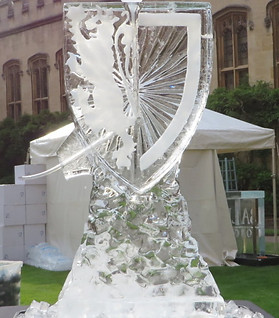top of page

## RESEARCH

Over the last century, logical and computational phenomena, in addition to more traditional physical ones, have provided mathematics with significant inspirations and been studied intensively. Today, as a consequence, mathematical logic and theoretical computer science have become mature as academic disciplines. From my (or a mathematician's) point of view, however, the very concepts of logic and computation have not been formulated in a completely satisfactory manner (as mathematics) especially because they have been studied mostly by syntactic and/or synthetic methods; that is, something like topological spaces for topology (or the even more basic concept of sets for almost all traditional branches of mathematics) has been missing for logic and computation. My research is primarily concerned with this fundamental problem, and I aim to establish a mathematical, in particular semantic and analytic, foundation of logic and computation, whence of (constructive) mathematics as well.

I believe that such an appropriate mathematical foundation would not only capture the abstract essence of logic and computation but also enable us to view unseen landscape in these fields, providing new mathematical techniques to solve open problems and/or conceptual ideas to develop further theories. For instance, my game-semantic approach to mathematical foundations of logic and computation has solved a long-standing open problem in constructive mathematics: the consistency of intensional Martin-Löf type theory with formal Church's thesis.

I am also interested in category theory, more broadly algebra, and its interplay with logic and computation, known as categorical logic. My current focus on these fields is to generalise categorical algebra so that it corresponds to the generalisation of propositional logic to predicate logic.

In addition, the recent advent of homotopy type theory has brought a novel, topological viewpoint into these fields. I am especially interested in such an interaction between logic, computation, category theory and topology. Out of this interaction, I aim to explore new computational foundations of topology and conversely topological structures in logic and computation.

Currently, I am about to complete my recent work on the unity of logic in terms of categorical algebra, proof theory and game semantics.bottom of page